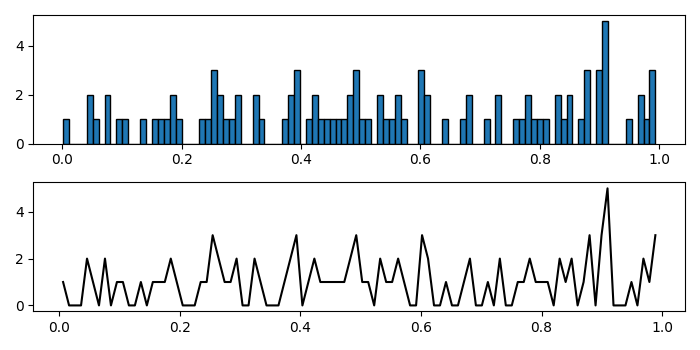# How to plot a line graph from histogram data in Matplotlib?

To plot a line graph from histogram data in matplotlib, we use numpy histogram method to compute the histogram of a set of data.

## Steps

• Add a subplot to the current figure, nrows=2, ncols=1 and index=1.

• Use numpy histogram method to get the histogram of a set of data.

• Plot the histogram using hist() method with edgecolor=black.

• At index 2, use the computed data (from numpy histogram). To plot them, we can use plot() method.

• To display the figure, use show() method.

## Example

import numpy as np
from matplotlib import pyplot as plt
plt.rcParams["figure.figsize"] = [7.00, 3.50]
plt.rcParams["figure.autolayout"] = True
plt.subplot(211)
data = np.array(np.random.rand(100))
y, binEdges = np.histogram(data, bins=100)
plt.hist(data, bins=100, edgecolor='black')
plt.subplot(212)
bincenters = 0.5 * (binEdges[1:] + binEdges[:-1])
plt.plot(bincenters, y, '-', c='black')
plt.show()

## Output